International
Tables for
Crystallography
Volume G
Definition and exchange of crystallographic data
Edited by S. R. Hall and B. McMahon

International Tables for Crystallography (2006). Vol. G. ch. 3.7, pp. 199-201

## Section 3.7.2. Binary image data

H. J. Bernsteina*

aDepartment of Mathematics and Computer Science, Kramer Science Center, Dowling College, Idle Hour Blvd, Oakdale, NY 11769, USA
Correspondence e-mail: yaya@bernstein-plus-sons.com

### 3.7.2. Binary image data

| top | pdf |

The six categories that collectively define the relationship between the sequences of octets in arrays of binary data and the information in the images those octets represent are as follows:

 ARRAY_DATA group The image data (§3.7.2.1) ARRAY_DATA Array elements (§3.7.2.2) ARRAY_ELEMENT_SIZE Intensities (§3.7.2.3) ARRAY_INTENSITIES Organization and encoding of array data (§3.7.2.4) ARRAY_STRUCTURE ARRAY_STRUCTURE_LIST ARRAY_STRUCTURE_LIST_AXIS

#### 3.7.2.1. The image data

| top | pdf |

Data items in this category are as follows:

ARRAY_DATAThe bullet () indicates a category key. The arrow () is a reference to a parent data item.

Each value of the _array_data.data data item is a sequence of octets representing a binary image. _array_data.array_id and _array_data.binary_id, taken together, uniquely identify each image. The value of _array_data.array_id is a pointer to _array_structure.id to provide the relationship between the sequence of octets and the logical structure of the image. Since multiple images may have the same logical structure, the purpose of _array_data.binary_id is to ensure that each image has a unique identifier.

#### 3.7.2.2. Array elements

| top | pdf |

Data items in this category are as follows:

ARRAY_ELEMENT_SIZEThe bullet () indicates a category key. The arrow () is a reference to a parent data item.

The value of the _array_element_size.size data item is a size in metres of an image element (a pixel or voxel). The direction of the measurement is given in each dimension by _array_element_size.index. The array structure specifying the organization of the dimensions is referenced by the value of _array_element_size.array_id, which is a pointer to _array_structure.id. The value of _array_element_size.index is a pointer to _array_structure_list.index. For data organized into rectangular arrays of pixels or voxels, this gives the spatial dimensions of the individual image elements.

#### 3.7.2.3. Intensities

| top | pdf |

Data items in this category are as follows:

ARRAY_INTENSITIESThe bullet () indicates a category key. The arrow () is a reference to a parent data item.

The relationship between the data values for individual image elements and the number of incident photons can be complex. The data items in the ARRAY_INTENSITIES category provide information about this relationship. The value of _array_intensities.linearity states the type of relationship, and the values of _array_intensities.array_id and _array_intensities.binary_id identify the array structure and the image being discussed. The other items are used in different ways depending on the relationship. If the value of _array_intensities.linearity is raw, then the image elements hold uninterpreted raw data values from the detector, e.g. for calibration. If the value of _array_intensities.linearity is linear, then the count in an image element is proportional to the incident number of photons by the value of _array_intensities.gain. The standard uncertainty (estimated standard deviation) of the gain may be given in _array_intensities.gain_esd. The value used for this should be estimated from a good understanding of the physical characteristics of the experimental apparatus. If the value of _array_intensities.linearity is offset, then the value of _array_intensities.offset should be added to the image element value. If the value of _array_intensities.linearity is scaling, scaling_offset, sqrt_scaled or logarithmic_scaled, the necessary scaling factor is given by the value of _array_intensities.scaling. In all cases, the scaling factor is applied to the image element value before the other operations are applied. In the first case, only simple scaling is used. In the second case, the value of _array_intensities.offset is added after scaling. In the third case, the scaled value is squared. In the final case, 10 is taken to the power given by the scaled value.

#### 3.7.2.4. Organization and encoding of array data

| top | pdf |

Data items in these categories are as follows:

(a) ARRAY_STRUCTURE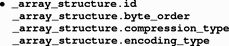(b) ARRAY_STRUCTURE_LIST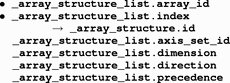(c) ARRAY_STRUCTURE_LIST_AXIS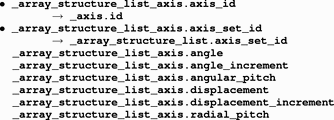The bullet () indicates a category key. The arrow () is a reference to a parent data item.

The data items in the ARRAY_STRUCTURE category show how the stream of octets in a binary image is to be reorganized into words of an appropriate size. Each possible encoding is identified by a value of _array_structure.id. In most cases, large images will have been compressed. The type of compression used is given by _array_structure.compression_type. Once a stream of octets has been decompressed, it can be organized into words. The type of each word is given by the value of _array_structure.encoding_type and the order of mapping octets onto words, most significant octet first (big-endian') or least significant octet first (little-endian'), is given by the value of _array_structure.byte_order.

The data items in the ARRAY_STRUCTURE_LIST category show how the list of words defined by the ARRAY_STRUCTURE category should be organized into image arrays. The value of _array_structure_list.array_id is a pointer to _array_structure.id. Each dimension (row, column, sheet etc.) of the image is identified by an index, counting from 1, given by _array_structure_list.index. The order of nesting of the indices is given by the values of _array_structure_list.precedence, with the index of precedence 1 varying most rapidly (i.e. having values stored sequentially). The direction of index change for increasing memory location is given by the value of _array_structure_list.direction. For a given index, the number of image elements in that direction is given by the value of _array_structure_list.dimension.

Data items in the ARRAY_STRUCTURE_LIST_AXIS category describe the physical settings of sets of axes for the centres of pixels that correspond to data points described in the ARRAY_STRUCTURE_LIST category.

In the simplest cases, the physical increments of a single axis correspond to the increments of a single array index. More complex organizations (e.g. spiral scans) may require coupled motions along multiple axes.

Note that a spiral scan uses two coupled axes, one for the angular direction and one for the radial direction. This differs from a cylindrical scan for which the two axes are not coupled into one set.

Multiple related axes are gathered together into sets. Each set is identified by the value of the axis set identifier, _array_structure_list_axis.axis_set_id, and each axis within a set is identified by the value of _array_structure_list_axis.axis_id. Each set given by a value of *.axis_set_id is linked to a corresponding value for _array_structure_list.axis_set_id to relate settings of the axes in the axis set to particular image elements in ARRAY_STRUCTURE_LIST.

If axes are all independent, no value need be given for _array_structure_list_axis.axis_set_id, which is then implicitly given the corresponding value of _array_structure_list_axis.axis_id. Each axis given by a value of _array_structure_list_axis.axis_id is linked to a corresponding value for _axis.id to provide a physical description of the axis. _array_structure_list_axis.axis_id and _array_structure_list_axis.axis_set_id together uniquely identify a row of data in an ARRAY_STRUCTURE_LIST_AXIS table.

For the remaining data items, there are two important cases to consider: axes that step by Euclidean distance and axes that step by angle. Fig. 3.7.2.1shows a portion of an array of image elements laid out on a rectangular grid. The starting point of an axis is specified in millimetres by the value of _array_structure_list_axis.displacement and the centre-to-centre distance between pixels is specified in millimetres by the value of _array_structure_list_axis.displacement_increment.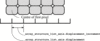Figure 3.7.2.1 | top | pdf |ARRAY_STRUCTURE_LIST specification of linearly organized image elements.

Fig. 3.7.2.2shows a portion of an array of image elements laid out in concentric cylinders. The starting point of the angular axis is specified in degrees by the value of _array_structure_list_axis.angle and the centre-to-centre angular distance between pixels is specified in degrees by the value of _array_structure_list_axis.angle_increment. The starting point of the radial axis is specified by the value of _array_structure_list_axis.displacement and the radial distance between cylinders of pixels is specified in millimetres by the value of _array_structure_list_axis.radial_pitch. Note that the image elements further from the centre are larger than the image elements closer to the centre.Figure 3.7.2.2 | top | pdf |ARRAY_STRUCTURE_LIST specification of constant-angle' image elements in a cylindrical scan. The angular and radial axes are independent. Note that outer-zone image elements are further apart, centre-to-centre, than inner-zone image elements.

Fig. 3.7.2.3shows a portion of a spiral scan array in which the angular and radial axes are coupled. This example represents a constant-velocity' scan, in which the size of the image elements does not depend on the distance from the centre. The starting point of the angular axis is again specified in degrees by the value of _array_structure_list_axis.angle, but the centre-to-centre distance between pixels is specified in millimetres by the value of _array_structure_list_axis.angular_pitch. The coupled radial axis is handled in much the same way as for the uncoupled radial axis in the cylindrical array.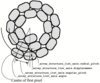Figure 3.7.2.3 | top | pdf |ARRAY_STRUCTURE_LIST specification of `constant-velocity' image elements in a cylindrical scan. The angular and radial axes are coupled. Note that outer-zone image elements are the same linear distance apart, centre-to-centre, as the inner-zone image elements.

These examples show some of the more common two-dimensional data structures. By coupling an additional axis not in the plane of the first two, regular three-dimensional arrays of data can be represented without additional tags. The categories in the DIFFRN group allow arrays of data to be associated with frames and thereby with time and/or wavelength. More general data structures, for example ones based on dope vectors or hash tables, would require the definition of additional tags, but any data structure (see Aho et al., 1987) that can be handled by a modern computer should be manageable within this framework.

### ReferencesAho, A. V., Hopcroft, J. E. & Ullman, J. D. (1987). Data structures and algorithms. Reading, MA: Addison–Wesley.Google Scholar